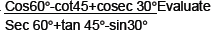# Introduction to Trigonometry-Important Questions

INTRODUCTION TO TRIGNOMETRY

HOTS
1.   Prove that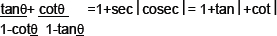2.   If tanA=n tanB and sin A =m sin B, prove that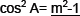n2 - 1
3.   Prove that following identity, where the angle involved is acute andgle for whhc the expression are expressed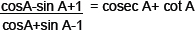using identity cosec2 A= 1+cot2A
4.   If x sin3|+ y cos3|=sin|cos| and xsin|=ycos|, prove x2+y2=1.
5.   Find the value of (sin°45- cos°45)
6.   If tan|+sin|=m and tan|- sin|=n, show that (m2-n2)=4√mn
7.   If cosec |-sin |=l and sec |- cos |=m, prove that l2m2(l2+m2+3)=1
8.   If sin A=1/2, then find the value ofcos A.
9.   If cosec |=3x and cot |=3/x. then find the value of (x2-1/x2)
10.   What is the value of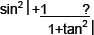11.   Given cosec|=4/3, calculate all other trigonometric ratios.
12.